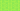Pricing# Residual Value: What is it and how to calculate it

Residual value is the projected value of a fixed asset when it’s no longer useful or after its lease term has expired. What is considered residual value varies across industries, but the core meaning is nevertheless retained. Keep reading to know more about the meaning of residual value, its benefits and how to calculate it.

## Introduction to Residual Value

Residual value refers to the estimated worth of an asset after the asset has fully depreciated.  Generally, the length of an asset’s lease period or useful life is inversely proportional to its residual value.

An asset’s residual value is determined based on the amount a company believes it will realise from the sale of the asset once its useful life or lease term ends. While different industries have different residual value formulas, the meaning of residual value does not change. It simply means what is left.

Residual value is viewed differently from various perspectives. In investments, for instance, residual value represents the difference between the cost of capital and profits. Whereas in capital projects budgeting, residual value is the amount receivable in exchange for an asset once the company no longer uses the asset or the revenue generated from it has become rather unpredictable.

Usually, the assumed residual value of an asset is zero.

## How to Calculate Residual Value

Various fields and industries differ in the way they calculate an asset’s residual value. However, the residual value of an asset is usually calculated from the estimated salvage value of that asset. The salvage value can be estimated using the comparable approach, i.e. based on the value of comparable assets in the market.

In the case of leasing, the lessor determines the residual value based on future estimates and past models.

Calculating residual value requires two figures namely, estimated salvage value and cost of asset disposal. Residual value equals the estimated salvage value minus the cost of disposing of the asset.

The residual value formula looks like this:

Residual value = (estimated salvage value) – (cost of asset disposal)

## Residual Value Example

Here, we’ll calculate the residual value of a piece of manufacturing equipment. Take that the manufacturing equipment cost \$40,000 and say the useful life is estimated at eight years. Let’s take \$5,000 as the estimated salvage value of the equipment when it’s disposed of as scrap metal after its useful life.

If it costs the company \$200 to move the equipment to the dumping ground, then the residual value of that asset is \$4,800 (\$5,000–\$200).

## Benefits of Residual Value

The benefits of residual value include the following:

• Determining Lease Rates: Residual value is one of the principal techniques a lessor uses to determine the amount a lessee will pay in regular lease costs. In a vehicle leasing deal, for instance, the monthly lease rate is determined using the residual value of the vehicle alongside tax and interest rate.

• Calculating Amortisation and Depreciation: Amortisation and depreciation calculations are important for accounting purposes. Now, residual value is one essential variable that companies use in calculating their yearly amortisation (for intangible assets) and depreciation (for tangible assets) figures. Companies have to factor in the residual value of their assets when they calculate the total depreciable sum to be used in their depreciation schedule.

Generally, it is ideal for companies to calculate their assets’ residual value at each year’s end. If and when the estimated residual value changes, such changes should be recorded accordingly.

It is worth remembering that companies owning several costly fixed assets such as vehicles, medical equipment and other heavy machinery may consider buying residual value insurance. This insurance helps to minimise asset-value risk by assuring the post-useful-life value of assets enjoying proper maintenance.

## We Can Help

GoCardless helps you automate payment collection, cutting down on the amount of admin your team needs to deal with when chasing invoices. Find out how GoCardless can help you with ad hoc payments or recurring payments.

GoCardless makes it easy to collect recurring payments